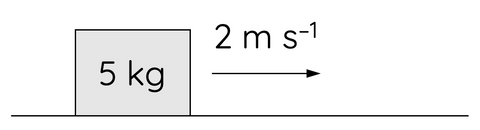# Law of Conservation of Momentum

This topic is part of the HSC Physics course under the section Momentum, Energy and Simple Systems.

### HSC Physics Syllabus

• conduct an investigation to describe and analyse one-dimensional (collinear) and two-dimensional interactions of objects in simple closed systems (ACSPH064)
• analyse quantitatively and predict, using the law of conservation of momentum Σmv_{\text{before}}= Σmv_{\text{after}} and, where appropriate, conservation of kinetic energy Σ1/2mv_{\text{before}}= Σ 1/2 mv_{\text{after}}, the results of interactions in elastic collisions (ACSPH066)

### What is Momentum?

In physics, momentum (represented by the symbol p) is a fundamental concept that describes the motion of an object. It is defined as the product of an object's mass and its velocity. In other words, the momentum of an object can be calculated using the equation:

$$p = mv$$

where:

• p is the momentum,
• m is the mass of the object, and
• v is its velocity.

Momentum is a vector quantity, meaning it has both magnitude (size) and direction.

The unit of momentum is kg m s-1.

The direction of momentum is the same as the direction of the velocity of the object.

Suppose a 5 kg mass is moving at 2 m s-1 to the right as shown.The momentum of this mass is given by

$$p = 5 \times 2$$

$$p = 10 \hspace{1mm} kg \hspace{1mm} m \hspace{1 mm} s^{-1}$$

The mass' momentum is 10 kg m s-1 to the right.

### The Law of Conservation of Momentum

Now, let's dive into the principle that describes how momentum interacts and changes in a system - the Law of Conservation of Momentum.

The law states that the total momentum of a closed system remains constant if no external forces act upon it. This is true for both collisions and explosions.

### What is a Closed System?

A "closed system," in physics, is one in which neither matter nor energy can enter or exit. But when discussing the law of conservation of momentum, we usually concern ourselves with systems closed to outside forces, even though some exchange of matter or energy may occur within the system. In these systems, the total momentum remains constant unless acted upon by an external net force.

### Example 1 – Crashing Cars

A 800 kg car was moving at 12 m s–1 east while a 2000 kg car was moving at 10 m s–1 west before they collided as shown. After the collision, both cars started to move together.What is the velocity of the cars?

The total momentum of the system before the cars collide is given by

$$p = m_1u_1 + m_2u_2$$

$$p = (800)(12) + (2000)(-10)$$

$$p = -10400 \hspace{1mm} kg \hspace{1mm} m \hspace{1mm} s^{-1}$$

By the law of conservation of momentum, the total momentum of the system after the cars collide will be equal to that before the collision:

$$-10400 = (m_1 + m_2)v$$

$$-10400 = (800 + 2000)v$$

$$v = -3.7 \hspace{1mm} m \hspace{1 mm} s^{-1}$$

Therefore, after collision the cars will move to the left at 3.7 m/s.

### Example 2 – Fired Gun with Recoil

A 5 kg rifle fires a 2 g bullet at 100 m s–1. What is the velocity of the rifle due to recoil?

Since the rifle and bullet were stationary, the total momentum of the system was zero before the rifle was fired.

By the law of conservation of momentum:

$$0 = m_1v_1 + m_2v_2$$

$$0 = (5)(v_1) + (0.002)(100)$$

$$v_1 = -0.04 \hspace{1mm} m \hspace{1mm} s^{-1}$$

Therefore, the rifle will experience a recoil backwards at 0.04 m/s.